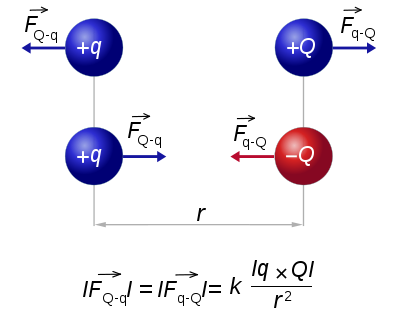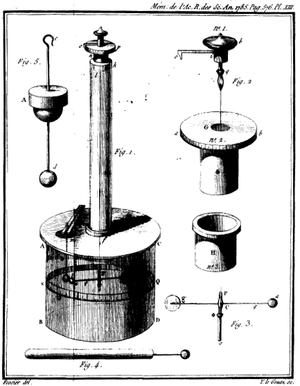# Coulomb's law

﻿
Coulomb's law

Coulomb's law or Coulomb's inverse-square law, is a law of physics describing the electrostatic interaction between electrically charged particles. It was first published in 1785 by French physicist Charles Augustin de Coulomb and was essential to the development of the theory of electromagnetism. Nevertheless, in 1767 Joseph Priestley of England conjectured that the force between charges varied as the inverse squared of the distance. In 1769, Scottish physicist John Robison announced that according to his measurements, the force of repulsion between two spheres with charges of the same sign varied as x-2.06. The dependence of the force between charged bodies upon both distance and charge had been discovered, but not published, in the early 1770s by Henry Cavendish of England, prior to Coulomb's works.

## Basic equationDiagram describing the basic mechanism of Coulomb's law; like charges repel each other and opposite charges attract each other.

The scalar form of Coulomb's law is an expression for the magnitude and sign of the electrostatic force between two idealized point charges, small in size compared to their separation. This force (F) acting simultaneously on point charges (q1) and (q2), is given by$F = k_\mathrm{e} \frac{q_1q_2}{r^2}$

where r is the separation distance and ke is a proportionality constant. A positive force implies it is repulsive, while a negative force implies it is attractive. The proportionality constant ke, called the Coulomb constant (sometimes called the Coulomb force constant), is related to defined properties of space and can be calculated based on the speed of light to be exactly:\begin{align} k_\mathrm{e} &= \frac{1}{4 \pi \varepsilon_0} = \frac{c^2 \ \mu_0}{4 \pi} = c^2 \cdot 10^{-7} \ \mathrm{H} \cdot \mathrm{m}^{-1}\\ &= 8.987\ 551\ 787\ 368\ 176\ 4 \times 10^9 \ \mathrm{N \cdot m^2 / C^{2}} \end{align}Coulomb's torsion balance

Coulomb's law states that: "The magnitude of the Electrostatics force of interaction between two point charges is directly proportional to the scalar multiplication of the magnitudes of charges and inversely proportional to the square of the distances between them."

In SI units, the meter is defined such that the speed of light in vacuum (or electromagnetic waves, in general), denoted c, is exactly 299,792,458 m·s−1, and the magnetic constant (μ0) is set at 4π × 10−7 H·m−1. In agreement with electromagnetic theory, requiring that${1 \over \mu _0 \varepsilon _0 }= c^2 ,$

the value for the electric constant (ε0) is derived to be ε0 = 1/(μ0c2) ≈ 8.85418782×10−12 F·m−1. In electrostatic units and Gaussian units, the unit charge (esu or statcoulomb) is defined in such a way that the Coulomb constant is 1 and dimensionless.

In the more useful vector-form statement, the force in the equation is a vector force acting on either point charge, so directed as to push it away from the other point charge; the right-hand side of the equation, in this case, must have an additional product term of a unit vector pointing in one of two opposite directions, e.g., from q1 to q2 if the force is acting on q2; the charges may have either sign and the sign of their product determines the ultimate direction of that force. Thus, the vector force pushing the charges away from each other (pulling towards each other if negative) is directly proportional to the product of the charges and inversely proportional to the square of the distance between them. The square of the distance part arises from the fact that the force field due to an isolated point charge is uniform in all directions and gets "diluted" with distance as much as the area of a sphere centered on the point charge expands with its radius.

The law of superposition allows this law to be extended to include any number of point charges, to derive the force on any one point charge by a vector addition of these individual forces acting alone on that point charge. The resulting vector happens to be parallel to the electric field vector at that point, with that point charge (or "test charge") removed.

Coulomb's law can also be interpreted in terms of atomic units with the force expressed in Hartrees per Bohr radius, the charge in terms of the elementary charge, and the distances in terms of the Bohr radius.

### Electric field

It follows from the Coulomb's Law that the magnitude of the electric field (E) created by a single point charge (q) at a certain distance (r) is given by:$E = {1 \over 4\pi\varepsilon_0}\frac{q}{r^2}.$

For a positive charge, the direction of the electric field points along lines directed radially away from the location of the point charge, while the direction is the opposite for a negative charge. The SI units of electric field are volts per meter or newtons per coulomb.

## Vector form

In order to obtain both the magnitude and direction of the force on a charge, q1 at position$\mathbf{r}_1$, experiencing a field due to the presence of another charge, q2 at position$\mathbf{r}_2$, the full vector form of Coulomb's law is required.$\mathbf{F} = {1 \over 4\pi\varepsilon_0}{q_1q_2(\mathbf{r}_1 - \mathbf{r}_2) \over |\mathbf{r}_1 - \mathbf{r}_2|^3} = {1 \over 4\pi\varepsilon_0}{q_1q_2 \over r^2}\mathbf{\hat{r}}_{21},$

where r is the separation of the two charges. This is simply the scalar definition of Coulomb's law with the direction given by the unit vector,$\mathbf{\hat{r}}_{21}$, parallel with the line from charge q2 to charge q1.

If both charges have the same sign (like charges) then the product q1q2 is positive and the direction of the force on q1 is given by$\mathbf{\hat{r}}_{21}$; the charges repel each other. If the charges have opposite signs then the product q1q2 is negative and the direction of the force on q1 is given by$-\mathbf{\hat{r}}_{21}$; the charges attract each other.

### System of discrete charges

The principle of linear superposition may be used to calculate the force on a small test charge, q, due to a system of N discrete charges:$\mathbf{F}(\mathbf{r}) = {q \over 4\pi\varepsilon_0}\sum_{i=1}^N {q_i(\mathbf{r} - \mathbf{r}_i) \over |\mathbf{r} - \mathbf{r}_i|^3} = {q \over 4\pi\varepsilon_0}\sum_{i=1}^N {q_i \over R_i^2}\mathbf{\hat{R}}_i,$

where qi and$\mathbf{r}_i$ are the magnitude and position respectively of the ith charge,$\mathbf{\hat{R}}_{i}$ is a unit vector in the direction of$\mathbf{R}_{i} = \mathbf{r} - \mathbf{r}_i$ (a vector pointing from charge qi to charge q), and Ri is the magnitude of$\mathbf{R}_{i}$ (the separation between charges qi and q).

### Continuous charge distribution

For a charge distribution an integral over the region containing the charge is equivalent to an infinite summation, treating each infinitesimal element of space as a point charge dq.

For a linear charge distribution (a good approximation for charge in a wire) where$\lambda(\mathbf{r^\prime})$ gives the charge per unit length at position$\mathbf{r^\prime}$, and$dl^\prime$ is an infinitesimal element of length,$dq = \lambda(\mathbf{r^\prime})dl^\prime$.

For a surface charge distribution (a good approximation for charge on a plate in a parallel plate capacitor) where$\sigma(\mathbf{r^\prime})$ gives the charge per unit area at position$\mathbf{r^\prime}$, and$dA^\prime$ is an infinitesimal element of area,$dq = \sigma(\mathbf{r^\prime})\,dA^\prime.\,$

For a volume charge distribution (such as charge within a bulk metal) where$\rho(\mathbf{r^\prime})$ gives the charge per unit volume at position$\mathbf{r^\prime}$, and$dV^\prime$ is an infinitesimal element of volume,$dq = \rho(\mathbf{r^\prime})\,dV^\prime.$

The force on a small test charge$q^\prime$ at position$\mathbf{r}$ is given by$\mathbf{F} = {q^\prime \over 4\pi\varepsilon_0}\int dq {\mathbf{r} - \mathbf{r^\prime} \over |\mathbf{r} - \mathbf{r^\prime}|^3}.$

### Graphical representation

Below is a graphical representation of Coulomb's law, when q1q2 > 0. The vector$\mathbf{F}_1$ is the force experienced by q1. The vector$\mathbf{F}_2$ is the force experienced by q2. Their magnitudes will always be equal. The vector$\mathbf{r}_{21}$ is the displacement vector between two charges (q1 and q2).A graphical representation of Coulomb's law.

## Electrostatic approximation

In either formulation, Coulomb’s law is fully accurate only when the objects are stationary, and remains approximately correct only for slow movement. These conditions are collectively known as the electrostatic approximation. When movement takes place, magnetic fields that alter the force on the two objects are produced. The magnetic interaction between moving charges may be thought of as a manifestation of the force from the electrostatic field but with Einstein’s theory of relativity taken into consideration.

### Atomic forces

Coulomb's law holds even within the atoms, correctly describing the force between the positively charged nucleus and each of the negatively charged electrons. This simple law also correctly accounts for the forces that bind atoms together to form molecules and for the forces that bind atoms and molecules together to form solids and liquids.

## Table of derived quantities

Particle property Relationship Field property
Vector quantity
 Force (on 1 by 2)$\mathbf{F}_{21}= {1 \over 4\pi\varepsilon_0}{q_1 q_2 \over r^2}\mathbf{\hat{r}}_{21} \$$\mathbf{F}_{21}= q_1 \mathbf{E}_{21}$
 Electric field (at 1 by 2)$\mathbf{E}_{21}= {1 \over 4\pi\varepsilon_0}{q_2 \over r^2}\mathbf{\hat{r}}_{21} \$
Relationship$\mathbf{F}_{21}=-\mathbf{\nabla}U_{21}$$\mathbf{E}_{21}=-\mathbf{\nabla}V_{21}$
Scalar quantity
 Potential energy (at 1 by 2)$U_{21}={1 \over 4\pi\varepsilon_0}{q_1 q_2 \over r} \$$U_{21}=q_1 V_{21} \$
 Potential (at 1 by 2)$V_{21}={1 \over 4\pi\varepsilon_0}{q_2 \over r}$

Wikimedia Foundation. 2010.

### Look at other dictionaries:

• Coulomb's law — Cou lomb s law (Physics) The law that the force exerted between two electric or magnetic charges is directly proportional to the product of the charges and inversely to the square of the distance between them. [Webster 1913 Suppl.] …   The Collaborative International Dictionary of English

• Coulomb's law — [ko͞o lämz′, ko͞o′lämz΄] n. Physics the statement that the electrostatic force between two charged bodies acts along the line between them, is directly proportional to the product of their magnitudes, and is inversely proportional to the square… …   English World dictionary

• Coulomb's law — Elect. the principle that the force between two point charges acts in the direction of the line between them and is directly proportional to the product of their electric charges divided by the square of the distance between them. [1850 55; after …   Universalium

• Coulomb’s law — Kulono dėsnis statusas T sritis fizika atitikmenys: angl. Coulomb’s law vok. Coulombsches Gesetz, n rus. закон Кулона, m pranc. loi de Coulomb, f …   Fizikos terminų žodynas

• Coulomb's Law — noun a fundamental principle of electrostatics; the force of attraction or repulsion between two charged particles is directly proportional to the product of the charges and inversely proportional to the distance between them; principle also… …   Useful english dictionary

• Coulomb's law — noun the fundamental law of electrostatics the force between two point charges is proportional to the product of their charges, and inversely proportional to the square of the distance between them …   Wiktionary

• Coulomb's law — [ ku:lɒmz] noun Physics a law stating that like electric charges repel and opposite charges attract, with a force proportional to the product of the charges and inversely proportional to the square of the distance between them …   English new terms dictionary

• Coulomb's Law — /ˈkulɒmz ˌlɔ/ (say koohlomz .law) noun (in physics) the principle that the attractive or repulsive force between two electrically charged bodies, or magnetic poles, is proportional to the product of the charges, or pole strengths, and inversely… …   Australian English dictionary

• Coulomb's law — noun Date: 1854 a statement in physics: the force of attraction or repulsion acting along a straight line between two electric charges is directly proportional to the product of the charges and inversely to the square of the distance between them …   New Collegiate Dictionary

• Coulomb's law — the force of attraction or repulsion between two electrified bodies is proportional directly to the quantities of electric charge, and inversely as the square of their distance apart …   Medical dictionary